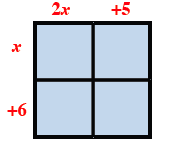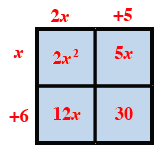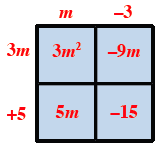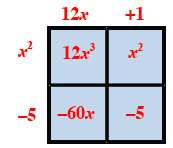Home > INT2 > Chapter 3 > Lesson 3.1.4 > Problem3-44

3-44.

Use an area model to multiply each of the following expressions. Write each expression both as a sum and as a product. Homework Help ✎

1. $(2x+5)(x+6)$

Draw a rectangular area model and label the length and width.Fill in the area model by multiplying the length and width of each box.

Write an equation showing the product is the sum of the parts.
Area as a sum: 2x2 + 12x + 5x + 30 or 2x2 + 17x + 30
Area as a product: (2x + 5)(x + 6)

1. $(m-3)(3m+5)$

See the help for part (a).Area as product: (m − 3)(3m + 5)
Area as sum: 3m2 − 4m − 15

1. $(12x+1)(x^2-5)$

See the help for part (a).1. $(3-5y)(2+y)$

See the help for part (a).